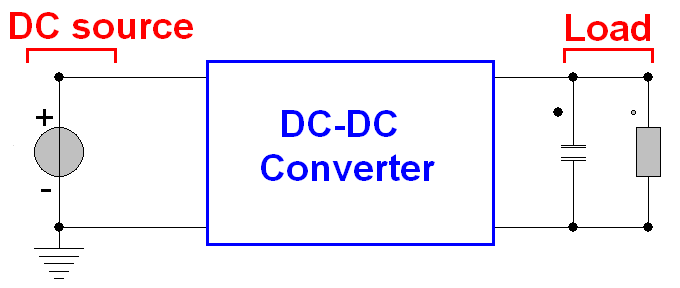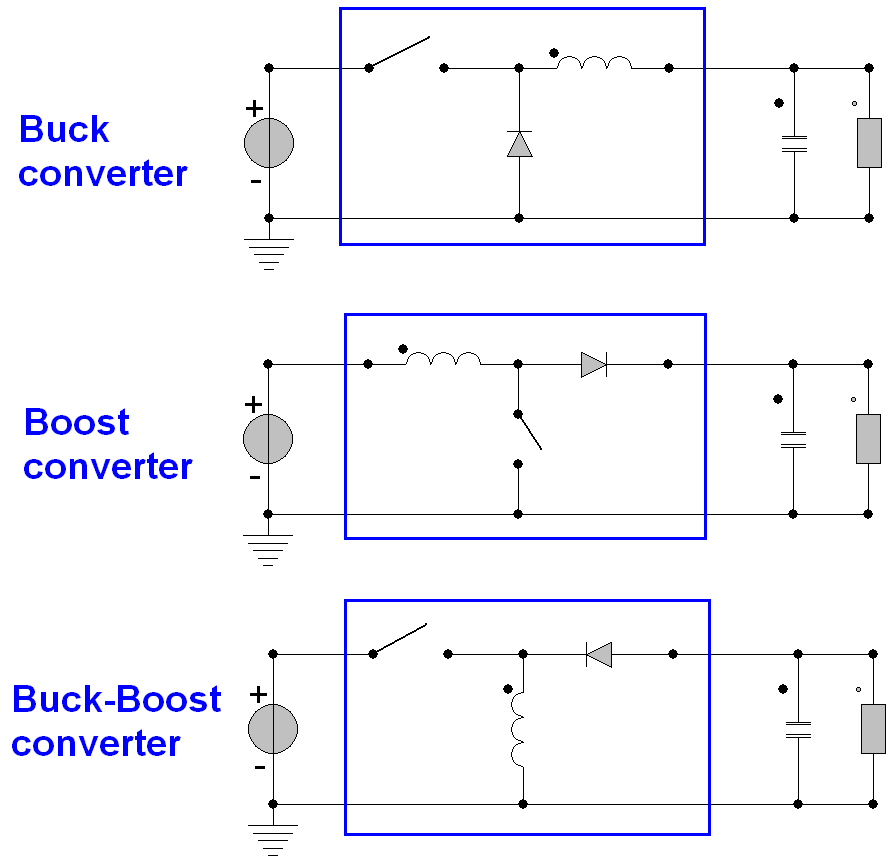## DC-DC converter

DC-DC converter is used to convert the voltage level of one DC source to another. The following picture shows the basic configuration for a DC-DC converter. The goal is to change the voltage level to the circuit load if the DC source doesn�t provide an expected voltage level.DC-DC converter is an electric circuit. Depending on the design of DC-DC converter, the output voltage level can be lower or higher than the DC source. If we want to lower the output voltage level of the DC-DC converter, the Buck converter will be a good choice. If we want to heighten its output voltage level, we shall choose the Boost converter. Moreover, according the parameters of the DC-DC converter, the output voltage lever can be much higher than the DC source or down to zero. In this example, we will show how to configure the buck and boost converters.

The steps are: (1) Find one DC source and set the electric ground (2) Give one resistor and one capacitor in parallel connection as the circuit load (3) Give one inductor, one diode and one switch for building a DC-DC convert.